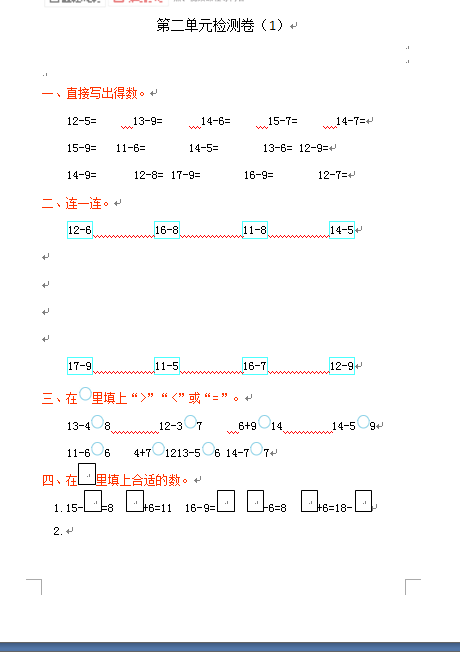12-5=　　　13-9=　　　14-6=　　　15-7=　　　14-7=

15-9=      11-6=　　      14-5=　　      13-6=  12-9=

14-9=　　    12-8=  17-9=　　      16-9=　　      12-7=

【DOC文档4页】小学人教版数学一年级下册第二单元检测卷（1）含答案A4电子版资料_可直接打印_会员免费下载##### 评论信息